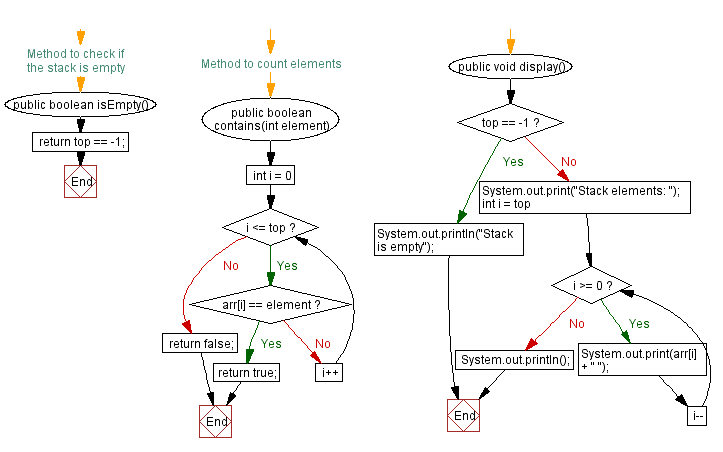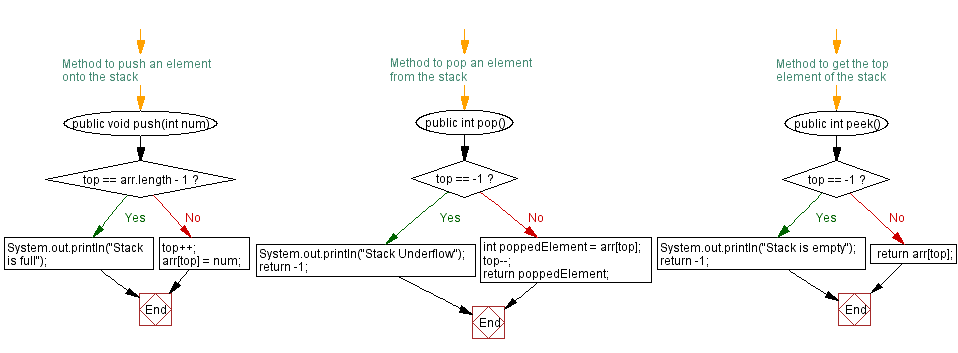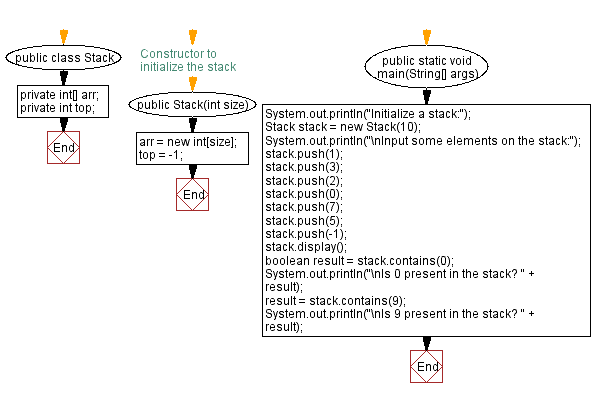# Java: Check if an element is present or not in a stack

## Java Stack: Exercise-8 with Solution

Write a Java program to implement a stack that checks if a given element is present or not in the stack.

Sample Solution:

Java Code:

``````import java.util.Scanner;
public class Stack {
private int[] arr;
private int top;

// Constructor to initialize the stack
public Stack(int size) {
arr = new int[size];
top = -1;
}

// Method to push an element onto the stack
public void push(int num) {
if (top == arr.length - 1) {
System.out.println("Stack is full");
} else {
top++;
arr[top] = num;
}
}

// Method to pop an element from the stack
public int pop() {
if (top == -1) {
System.out.println("Stack Underflow");
return -1;
} else {
int poppedElement = arr[top];
top--;
return poppedElement;
}
}

// Method to get the top element of the stack
public int peek() {
if (top == -1) {
System.out.println("Stack is empty");
return -1;
} else {
return arr[top];
}
}

// Method to check if the stack is empty
public boolean isEmpty() {
}

// Method to count elements
public boolean contains(int element) {
for (int i = 0; i <= top; i++) {
if (arr[i] == element) {
return true;
}
}
return false;
}

public void display() {
if (top == -1) {
System.out.println("Stack is empty");
} else {
System.out.print("Stack elements: ");
for (int i = top; i >= 0; i--) {
System.out.print(arr[i] + " ");
}
System.out.println();
}
}

public static void main(String[] args) {
System.out.println("Initialize a stack:");
Stack stack = new Stack(10);
System.out.println("\nInput some elements on the stack:");
stack.push(1);
stack.push(3);
stack.push(2);
stack.push(0);
stack.push(7);
stack.push(5);
stack.push(-1);
stack.display();
boolean result = stack.contains(0);
System.out.println("\nIs 0 present in the stack? " + result);
result = stack.contains(9);
System.out.println("\nIs 9 present in the stack? " + result);
}
}
```
```

Sample Output:

```Initialize a stack:

Input some elements on the stack:
Stack elements: -1 5 7 0 2 3 1

Is 0 present in the stack? true

Is 9 present in the stack? false
```

Flowchart:Live Demo:

Java Code Editor:

Improve this sample solution and post your code through Disqus

Java Stack Previous: Count all the elements in a stack.
Java Stack Exercises Next: Remove duplicates from a given stack.

What is the difficulty level of this exercise?

Test your Programming skills with w3resource's quiz.

﻿